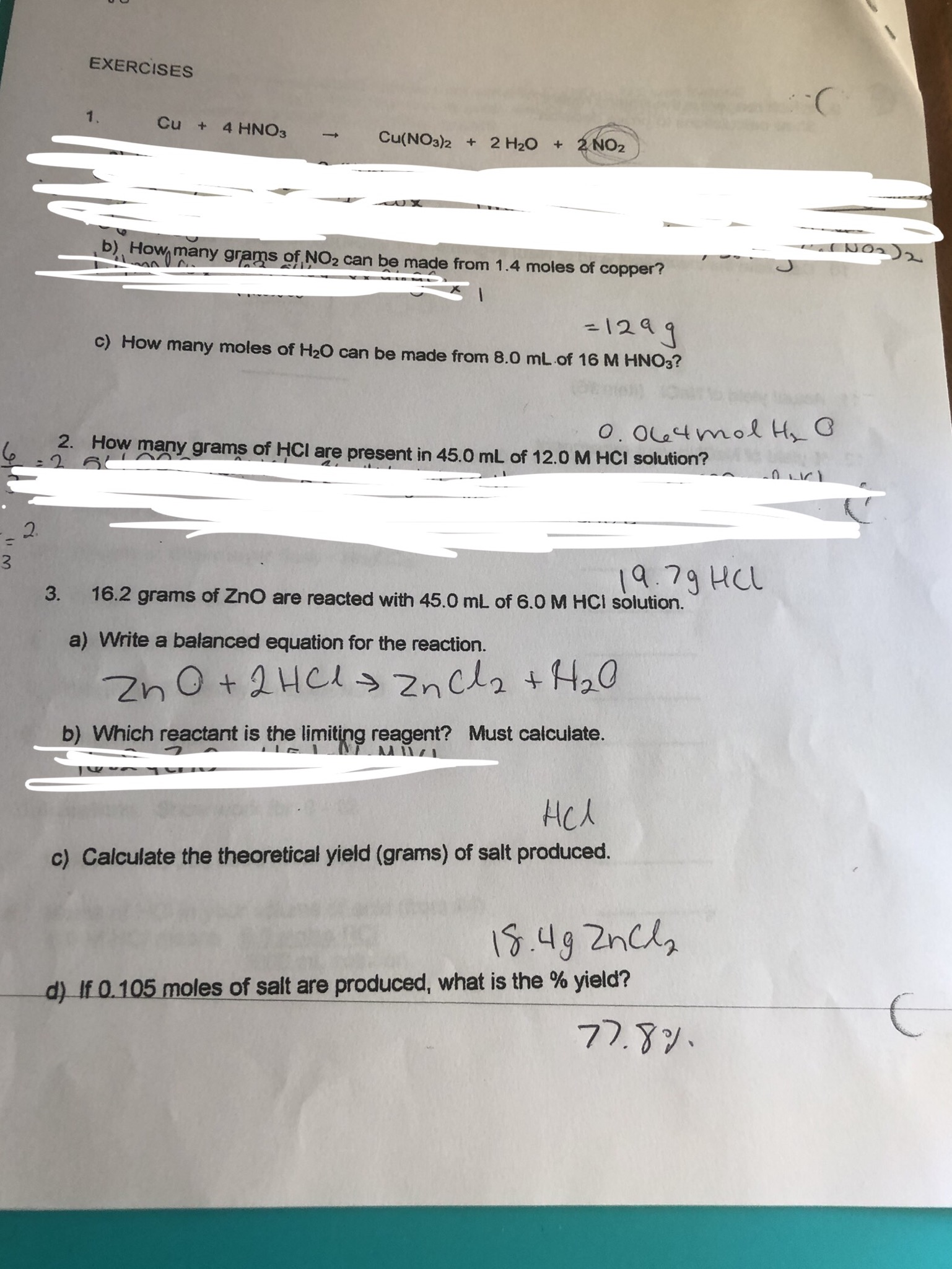# EXERCISESCu + 4 HNO3Cu(NO3)2+ 2 H20 +2NO2Howmany grams of NO2 can be made from 1.4 moles of copper?-1241c) How many moles of H20 can be made from 8.0 mL of 16 M HNO3?0. 0u4mol H G2.How many grams of HCI are present in 45.0 mL of 12.0 M HCI solution?26 AA231979 HCL16.2 grams of ZnO are reacted with 45.0 mL of 6.0 M HCI solution.3.a) Write a balanced equation for the reaction.b) Which reactant is the limiting reagent? Must calculate.HcAc) Calculate the theoretical yield (grams) of salt produced.IS.49 2ncld) If 0.105 moles of salt are produced, what is the % yield?77.79.

Question
51 views

Question 1Chelp_outlineImage TranscriptioncloseEXERCISES Cu + 4 HNO3 Cu(NO3)2+ 2 H20 +2NO2 Howmany grams of NO2 can be made from 1.4 moles of copper? -1241 c) How many moles of H20 can be made from 8.0 mL of 16 M HNO3? 0. 0u4mol H G 2. How many grams of HCI are present in 45.0 mL of 12.0 M HCI solution? 2 6 AA 2 3 1979 HCL 16.2 grams of ZnO are reacted with 45.0 mL of 6.0 M HCI solution. 3. a) Write a balanced equation for the reaction. b) Which reactant is the limiting reagent? Must calculate. HcA c) Calculate the theoretical yield (grams) of salt produced. IS.49 2ncl d) If 0.105 moles of salt are produced, what is the % yield? 77.79. fullscreen
check_circle

Step 1

Concentration of nitric acid = 16M = 16mol/L.

Volume of nitri...

### Want to see the full answer?

See Solution

#### Want to see this answer and more?

Solutions are written by subject experts who are available 24/7. Questions are typically answered within 1 hour.*

See Solution
*Response times may vary by subject and question.
Tagged in

### General Chemistry